Question

# The variable Z has a standard normal distribution. The probability P(-1.27 < Z < 2.19) is:...

1. The variable Z has a standard normal distribution. The probability P(-1.27 < Z < 2.19) is:

 a. 0.9852 b. 0.102 c. 0.883 d. 0.8832

QUESTION 6

1. The probability P(-1.45<= Z <= 0) is:

 a. 0.9929 b. 0.0735 c. 0.4265 d. 0.5071

3

1. If P(Z > z) = 0.7881, then the z-score is:

 a. 0.8 b. -0.8 c. 0.58 d. -0.58#### Earn Coins

Coins can be redeemed for fabulous gifts.

Similar Homework Help Questions
• ### Find the following z values for the standard normal variable Z. (You may find it useful...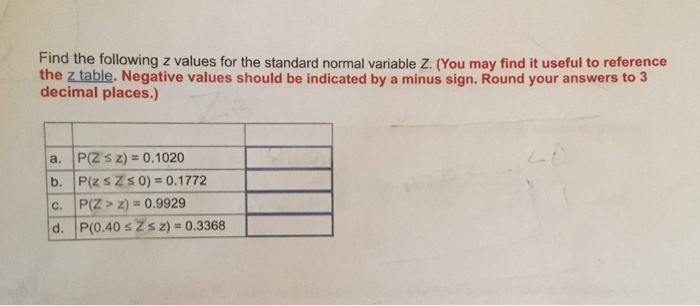Find the following z values for the standard normal variable Z. (You may find it useful to reference the z table. Negative values should be indicated by a minus sign. Round your answers to3 decimal places.) a. P(Z s z)-0.1020 b. P(z s Z s 0)-0.1772 c. P(Z> z) 0.9929 d. P(0.40 sZsz)- 0.3368

• ### Find the following z values for the standard normal variable Z. (You may find it useful...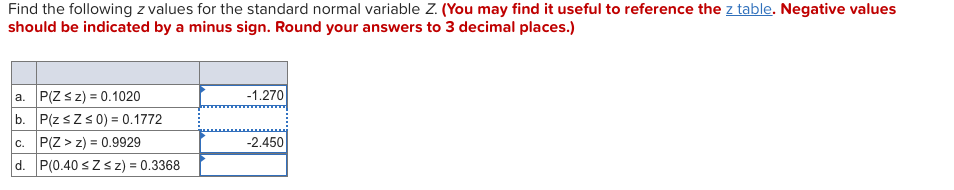Find the following z values for the standard normal variable Z. (You may find it useful to reference the z table. Negative values should be indicated by a minus sign. Round your answers to 3 decimal places.) a. P(Z S z) 0.1020 b. P(z s Zs0) 0.1772 c. P(Z> z)-0.9929 d. P(0.40 sZz)0.3368 1.270 2.450

• ### For a standard normal distribution, find: P(z 2.19) Points possible: 1 This is attempt 1 of...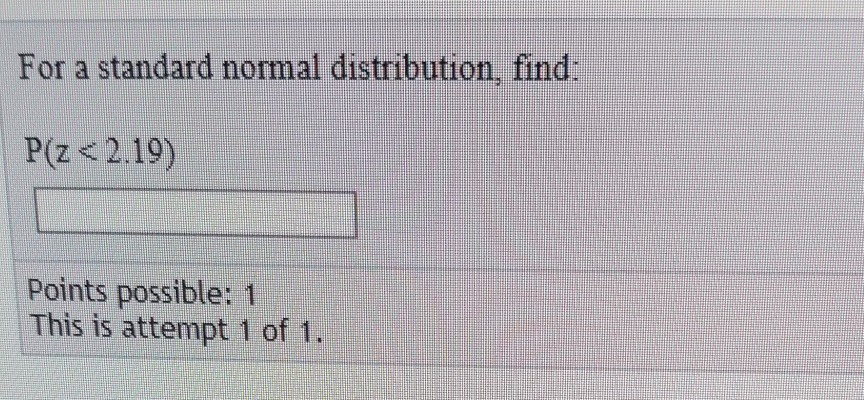For a standard normal distribution, find: P(z 2.19) Points possible: 1 This is attempt 1 of 1

• ### Standard Normal distribution. With regards to a standard normal distribution complete the following: (a) Find P(Z...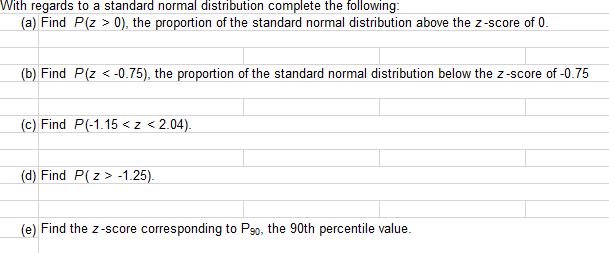Standard Normal distribution. With regards to a standard normal distribution complete the following: (a) Find P(Z > 0), the proportion of the standard normal distribution above the z-score of 0. (b) Find P(Z <-0.75), the proportion of the standard normal distribution below the Z-score of -0.75 (c) Find P(-1.15<z <2.04). (d) Find P(Z > -1.25). (e) Find the Z-score corresponding to Pso, the 90th percentile value.

• ### Let z be a random variable with a standard normal distribution. Calculate the indicated probability P(−1.15≤...

Let z be a random variable with a standard normal distribution. Calculate the indicated probability P(−1.15≤ z ≤1.55)P(−1.15≤ z ≤1.55).

• ### Find the following probabilities for a standard normal variable, Z 1) P(Z<-1.27) 2) P(-2.03<Z<3.49) 3) P(Z>1.74)...

Find the following probabilities for a standard normal variable, Z 1) P(Z<-1.27) 2) P(-2.03<Z<3.49) 3) P(Z>1.74) 4)P(Z<0.17) B. Find z if we know that the area to the left of z (under the normal curve) is 0.9265.

• ### 2. Random variable Z has the standard normal distribution. Find the following probabilities a): P[Z >...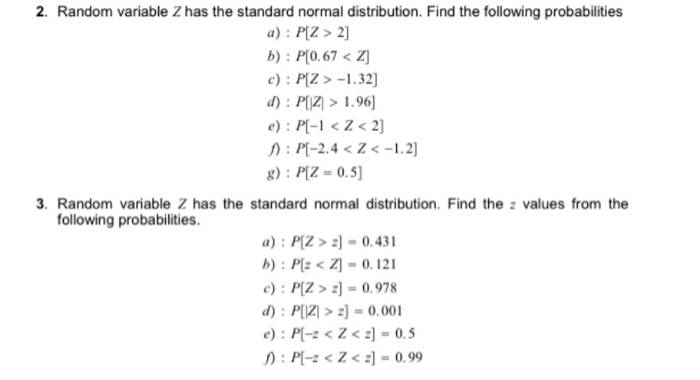2. Random variable Z has the standard normal distribution. Find the following probabilities a): P[Z > 2] b) : P[0.67 <z c): P[Z > -1.32] d): P(Z > 1.96] e): P[-1 <Z <2] : P[-2.4 < Z < -1.2] g): P[Z-0.5) 3. Random variable 2 has the standard normal distribution. Find the values from the following probabilities. a): P[Z > 2) - 0.431 b): P[:<] -0.121 c): P[Z > 2] = 0.978 d): P > 2] -0.001 e): P[- <Z...

• ### Let z be a random variable with a standard normal distribution. Find the indicated probability below...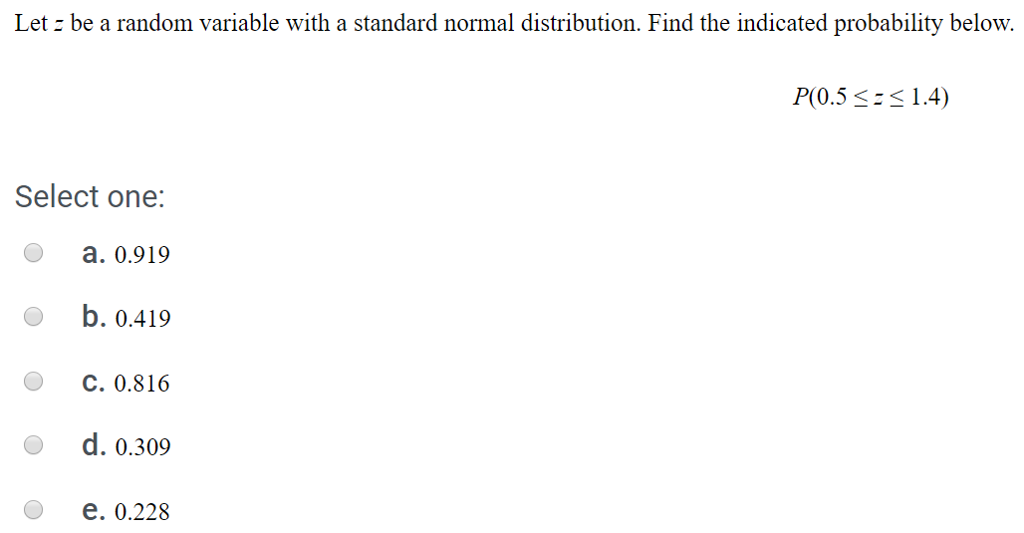Let z be a random variable with a standard normal distribution. Find the indicated probability below P(0.5 1.4) Select one: a. 0.919 li), о.419 O b. C. 0.816 d. 0.309 e. 0.228 o

• ### IS Find the following probability for the standard normal random variable z. a. P(z<-1.02) b. P(z...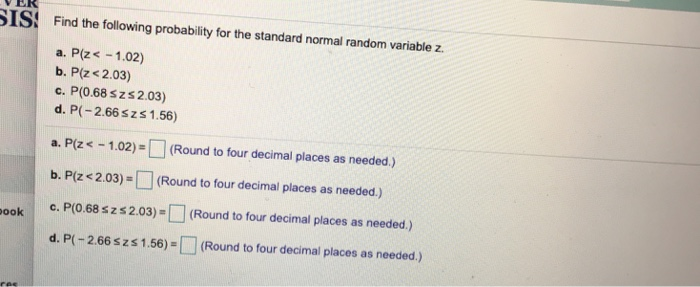IS Find the following probability for the standard normal random variable z. a. P(z<-1.02) b. P(z <2.03) c. P(0.68 szs2.03) d. P(-2.66szs1.56) a. P(z -1.02)(Round to four decimal places as needed.) b. Pize 2.03)=[] (Round to four decimal places as needed.) ook c. P(0.68 szs2.03) (Round to four decimal places as needed.) d.P(-2.66 s zs 1.56) = [□ (Round to four decimal places as needed.)

• ### if z is a standard normal variable find the probability that (p(-0.73) < z <2.27 If...

if z is a standard normal variable find the probability that (p(-0.73) < z <2.27 If z is a standard normal variable find the probability that p(z < 2.01)